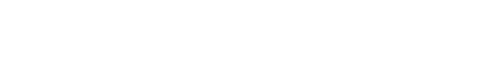How to do linear regression in SQL | Mode

# How to do linear regression in SQL# How to do linear regression in SQL

Why we wrote this whitepaper

Linear regression is one of the most powerful tools in a data scientist’s pocket. In this intermediate-advanced tutorial, we’ll show you how you can get a simple regression analysis done in pure SQL with relatively little pain.

In this guide, we’ll cover

• Why you would want to do regression in SQL and how to do it in Mode
• How you can test for statistical significance
• How to validate your inputs
• Why simple statistical methods are useful52% of Fortune 500 companies rely on Mode